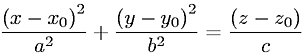Equations > Geometry > Curves and Shapes > Equation of an Elliptic Paraboloid

### Equation of an Elliptic ParaboloidLatex Code:

MathML Code:

 $\frac{\left(x-{x}_{0}\right)}^{2}{a}^{2}+\frac{\left(y-{y}_{0}\right)}^{2}{b}^{2}=\frac\left(z-{z}_{0}\right)c$

MathType 5.0: